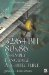Speed FreakThe code size would increase but using a vector table such as follows would allow you to unroll your (remainder) loops . With normal code, four states would be required but for MMX all eight would be best.

mov      eax,ecx           ; Get Width         and      eax,0000111b         jmp      \$SetTbl[eax*4]     ; At bottom of assembly source file insert the vector table so ; it doesn't interfere with your memory caches.             Align 16 \$SetTbl:dd       \$SetQ           ; (n mod 8) = 0         dd       \$Set1           ; (n mod 4) = 1         dd       \$Set2           ; (n mod 4) = 2         dd       \$Set3           ; (n mod 4) = 3         dd       \$SetD           ; (n mod 4) = 0         dd       \$Set1           ; (n mod 4) = 1         dd       \$Set2           ; (n mod 4) = 2         dd       \$Set3           ; (n mod 4) = 332/64-Bit 80x86 Assembly Language Architecture
ISBN: 1598220020
EAN: 2147483647
Year: 2003
Pages: 191

Similar book on Amazon